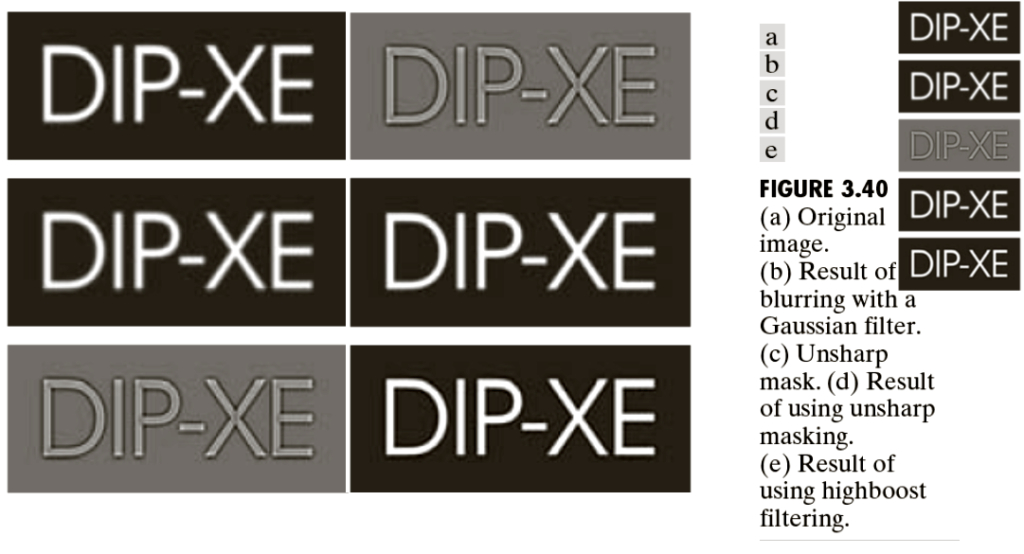# Fundamental of Spatial Filtering

### Fundamentals of Spatial Filtering

• Filtering unwanted frequency components.
• The term filter is borrowed from frequency domain processing
• accepting or rejecting certain frequency components
• Some non-linear filtering that cannot be done in frequency domain filter
• Spatial filters
• kernels
• templates
• windows

### Mechanics of Spatial Filtering

• Spatial filter consist of
• A neighborhood (typically a small rectangle)
• A predefined operation involving the neighborhood
• Filtering creates a new pixel at the same location as of original image but in the new image.
• A filtered (processed) image is generated as the filter visits each pixel in the input image.

### Mechanics of  Spatial Filtering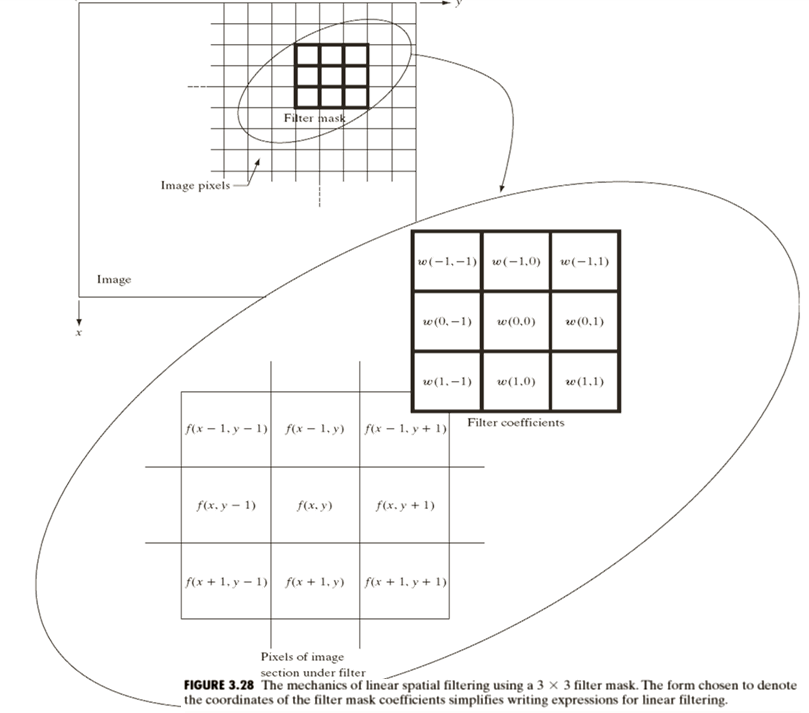### Mechanics of Spatial Filtering

• At any point x,y in the image the response g(x,y) of the filter is

g(x,y)=w(-1,-1)f(x-1,y-1) + w(-1,0)f(x-1,y) +w(-1,1)f(x-1,y+1)

+w(0,-1)f(x,y-1) + w(0,0)f(x,y) + w(0,1)f(x,y+1)

+w(1,-1)f(x+1,y-1) + w(1,0)f(x+1,y) + w(1,1)f(x+1,y+1)

### Filters Brief Classification in the book

• Spatial Filters
• Smoothing Spatial Filters (LPF)
• Smoothing Linear Filters
• Averaging Filter
• Weighted Average Filter
• Order-Statistic Filters (Non-Linear)
• Sharpening Spatial Filters (HPF)
• Laplacian Filter
• Unsharp Masking and Highboost Filtering

### Smoothing Spatial Filters

• Smoothing spatial filters are used for blurring and noise reduction in a digital image.
• Blurring is used in preprocessing tasks such as removal of small details from an image prior to object (large details) extraction.
• Two further types
• Smoothing Linear Filters
• Order-Statistic Filters (Non-Linear)

### Smoothing Linear Filters

• Averaging Filter
• Low Pass Filter
• Box filter
• Weighted Average Filter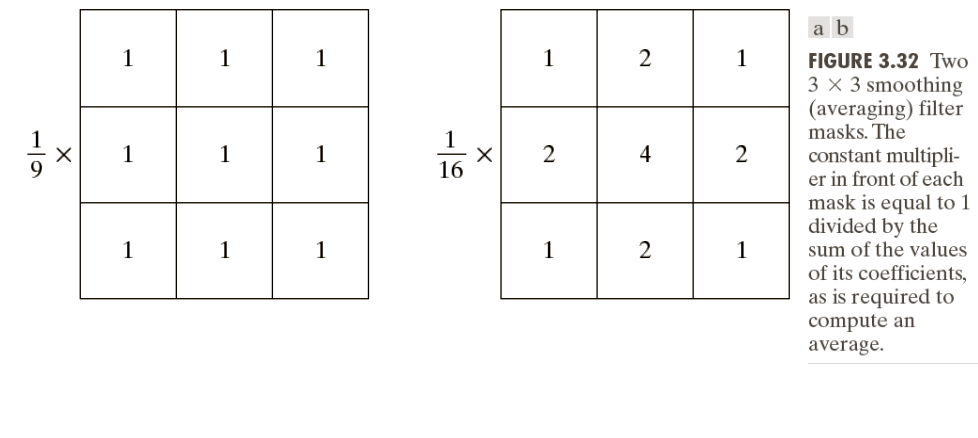### Smoothing Linear Filters

• High Frequency Component
• A term frequently used in subject of Digital Signal Processing
• Sharp Transition in some image is actually High Frequency Component and not always a desired and needed property in all portions of the image.
• Removal of irrelevant details from the image.

Smoothing Linear Filters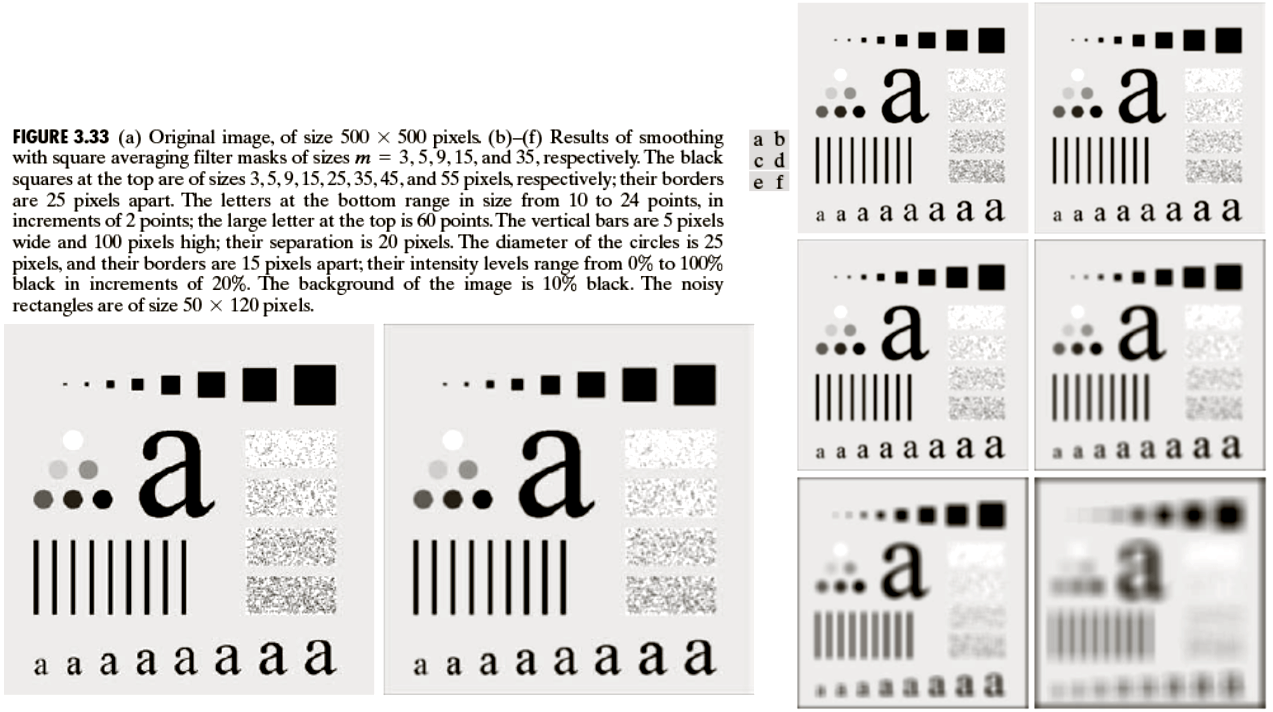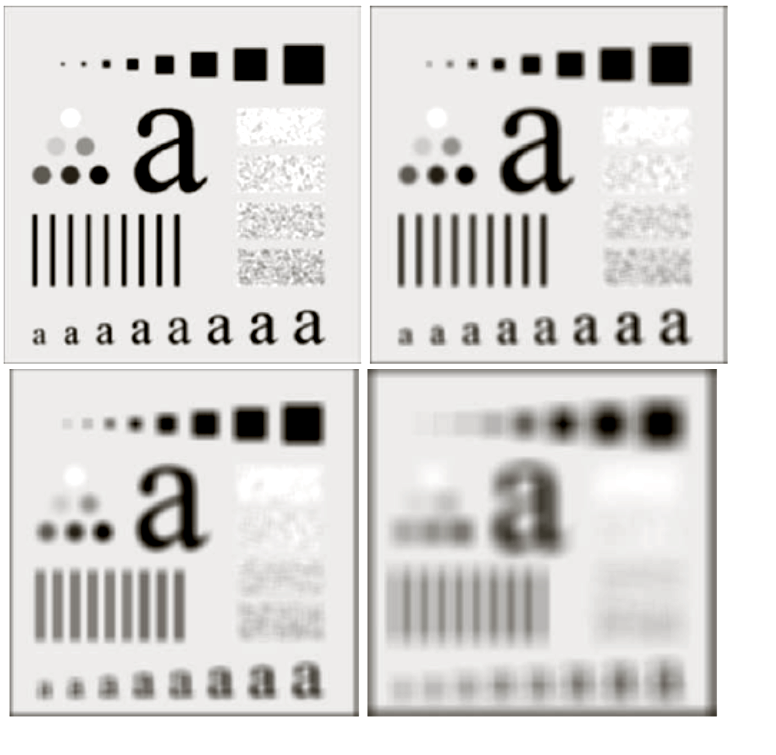### Smoothing Linear Filters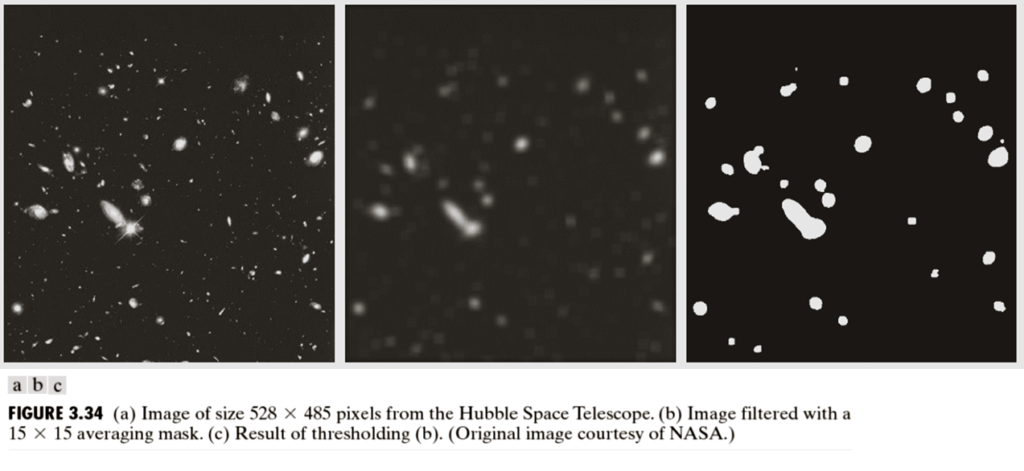### Order-Statistic Filters (Non-Linear)

• Response is based on ordering (ranking) the pixels contained in the image area along the filter and then determine the value of the center pixel with the value determined by the ranking result.
• Best known filter in this category is the median filter, which replaces the value of a pixel by the median of the intensity values in the neighborhood of that pixel.

Median Filters

• Median filters are quite popular because, for certain types of random noise, they provide excellent noise-reduction capabilities, with considerably less blurring than linear smoothing filters of similar size.
• They are particularly effective in the presence of impulse noise, also called salt-and-pepper noise.

### Median Filters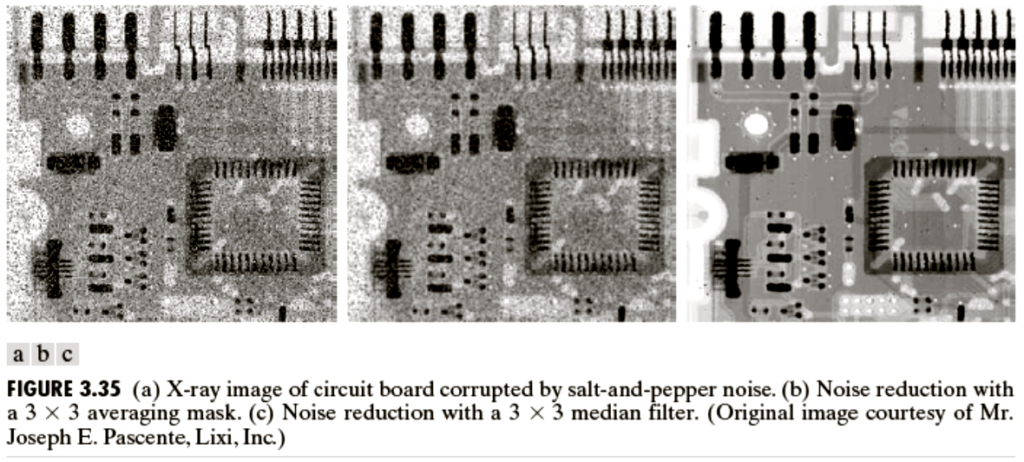### Median

• How median is calculated ?
• Assignment: Write a function in MATLAB that apply median filter on the input image. Do not use built-in commands.

### Sharpening Spatial Filter

• Principle objective of Sharpening is to highlight transitions in the intensity.
• Highlight edges
• These filters are derived using differentiation (derivative).
• Primary interest of the design of these filters is to achieve isotropic filters
• Isotropic filters are rotation invariant (rotating the image does not effect the result)
• Anisotropic (the opposite concept )

### Sharpening Spatial Filter

• Also called Laplacian Filters
• (Dervation in the book for details)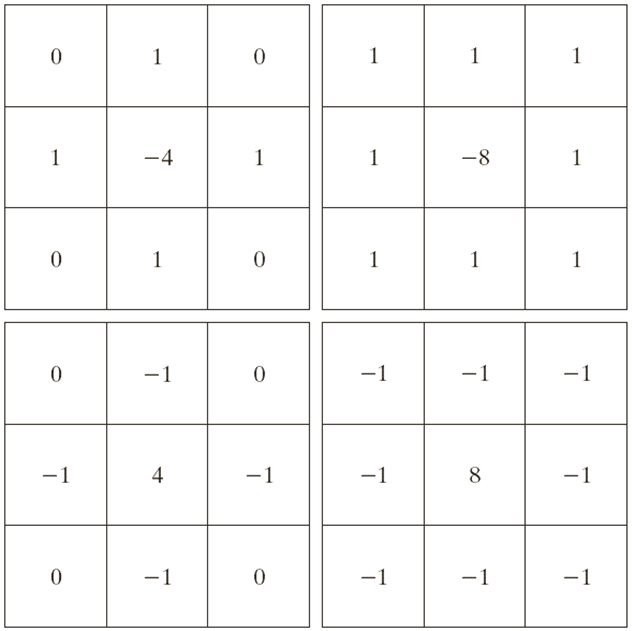### Sharpening Spatial Filter

• Drawback: Featureless background
• Filtered image is also called the Laplacian image
• Background features can be recovered by adding the filtered image to the original image if the Laplacian filter used has positive center coefficient. If the center coefficient is negative then we need to subtract the filtered image.
• g(x,y) = f(x,y) + c [▽2 f(x,y) ]

### Sharpening Spatial Filter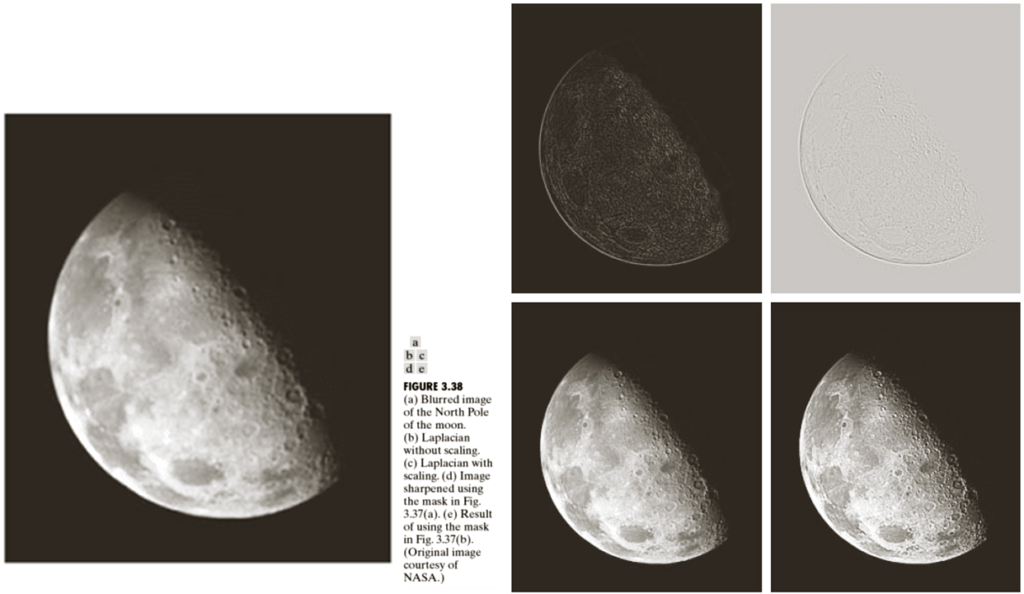### Unsharp Masking and Highboost Filtering

1.Blur the original image (using LPF)

2.Subtract the blurred image from the original ( the resulting difference is called the mask)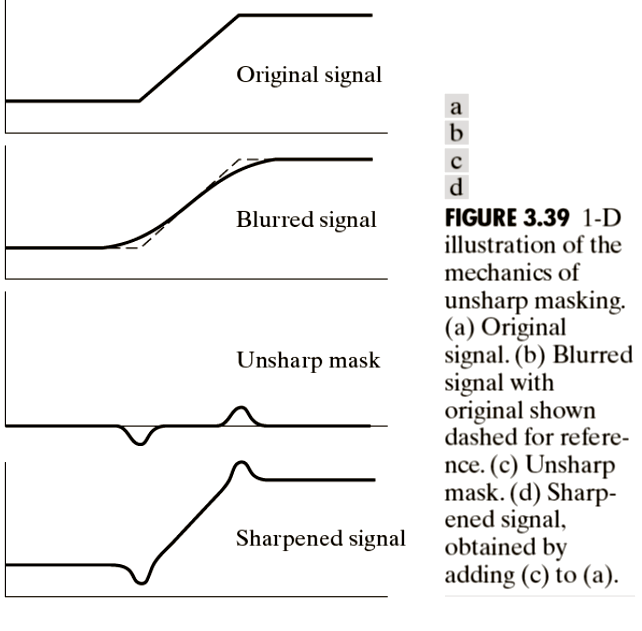### Unsharp Masking and Highboost Filtering

• g(x,y) = f(x,y) + k * gmask(x,y)
• Here gmask(x,y) is [Subtraction of blurred image from the original image]
• When k>1, the process is referred to as Highboost Filtering

### Unsharp Masking and Highboost Filtering